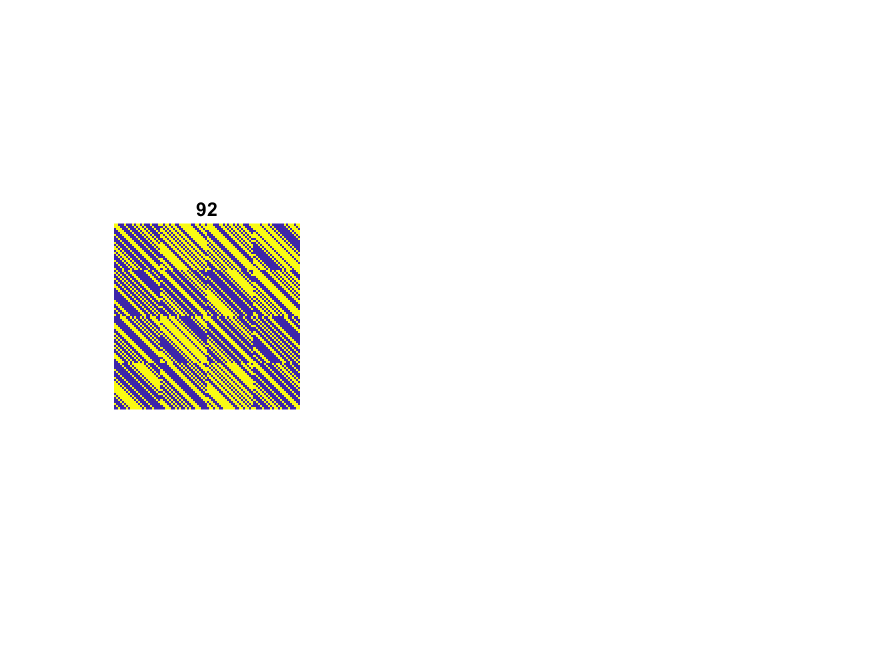Cleve’s Corner: Cleve Moler on Mathematics and ComputingScientific computing, math & more

I have just returned from the ICIAM2019 conference in Valencia, Spain. It was a huge conference -- 4,000+ attendees, dozens of prize and invited talks, hundreds of parallel minisympsia. I gave a talk in a two-part minisymposium organized by Nick Higham and Rob Corless. I outlined the first part of the talk in this blog a month ago. This post outlines the second part, which was about Hadamard matrices. Some of it is taken from a post in this blog five years ago.

Contents

The determinant of any matrix cannot be larger than the product of the length of its rows. This leads to Hadamard's inequality, for any $n$ by $n$ matrix, $A$, with elements +1 or -1,

$|\mbox{det}(A)| \le n^{n/2}$

A Hadamard Matrix is a square matrix $H$ with elements +1 or -1 whose rows (or columns) are mutually orthogonal, that is

$H^T H = nI$

A Hadamard matrix achieves equality in the Hadamard inequality. Conversely, any matrix achieving equality must be a Hadamard matrix.

$$H = \left( \begin{array}{rr} 1 & 1 \\ 1 & -1 \\ \end{array} \right)$$

Notice that

$|\mbox{det}(H)| = 2 = 2^{2/2}$

A direct search of all 3-by-3 matrices shows

$$A = \left( \begin{array}{rrr} 1 & 1 & 1 \\ 1 & -1 & 1 \\ 1 & 1 & -1 \end{array} \right)$$

and its permutations have the largest determinant.

But the rows of $A$ are not mutually orthogonal and

$|\mbox{det}(A)| = 4 < 3^{3/2} = 5.196$

We conclude no 3-by-3 Hadamard matrices exist.

$$H = \left( \begin{array}{rrrr} 1 & 1 & 1 & 1 \\ 1 & -1 & 1 & -1 \\ 1 & 1 & -1 & -1 \\ 1 & -1 & -1 & 1 \end{array} \right)$$

Notice

$|\mbox{det}(H)| = 16 = 4^{4/2}$

Existence

These three examples are special cases of this important fact: The order of a Hadamard matrix must be 1, 2, or a multiple of 4. But what about the reverse question, does a Hadamard matrix of order $n = 4k$ exist for every $k$? Nobody knows for sure. This is an important open mathematical problem.

Generation

It is easy to create Hadamard matrices of some sizes. If $H$ is any Hadamard matrix, then so is the block matrix

$$\left( \begin{array}{rr} H & H \\ H & -H \\ \end{array} \right)$$

You can start a recursion with the 1-by-1 matrix $H = 1$ and generate Hadamard matrices whose orders are powers of two. The MATLAB function hadamard can also start with a 12-by-12 or a 20-by-20 $H$. Here is what you get. Yellow is +1 and blue is -1.Baumert92

I was present at a milestone in the history of Hadamard matrices, By 1961 it was known how to construct Hadamard matrices for all orders $n \le 100$ that are multiples of four, except $n = 92$. In 1961 I had a summer job at JPL, Caltech's Jet Propulsion Lab. My office was in a temporary trailer and my trailer mate was an algebraist named Len Baumert. Len proudly showed me a color graphic that he had just made with an hour-long computation on JPL's main-frame and that he was proposing for the cover of Scientific American. It was a 92-by-92 matrix made up from 23-by-23 blocks of alternating light and dark cells representing +1 and -1. The graphic didn't make the cover of Scientific American, but I kept my copy for a long time.

Len had filled in the last value of $n$ less than 100. His paper with his colleagues Sol Golomb, a professor at USC, and Marshall Hall, Jr., a professor at Caltech, was published in the prestigious Bulletin of the AMS. It turns out that I had taken a course on difference sets, the mathematics involved in generating the matrix, from Hall at Caltech.

Here is a MATLAB picture and code for Baumert92.References

Leonard Baumert, S. W. Golomb, and Marshall Hall, Jr, "Discovery of an Hadamard Matrix of Order 92", <http://dx.doi.org/10.1090%2FS0002-9904-1962-10761-7>, Bulletin of the American Mathematical Society 68 (1962), 237-238.

code

type Baumert92.m
function H = Baumert92
% Baumert92  Hadamard matrix of order 92.
% H = Baumert92

% Baumert, Golomb, and Hall, Bulletin AMS 68, 1962, 237-238.
% http://dx.doi.org/10.1090%2FS0002-9904-1962-10761-7

% First row of 23-by-23 circulants.

M = [ '+ + - - - + - - - + - + + - + - - - + - - - +'
'+ - + + - + + - - + + + + + + - - + + - + + -'
'+ + + - - - + + - + - + + - + - + + - - - + +'
'+ + + - + + + - + - - - - - - + - + + + - + +' ];

M = 2*(M(:,1:2:end)=='+')-1;

A = toeplitz([1 M(1,end:-1:2)],M(1,:));
B = toeplitz([1 M(2,end:-1:2)],M(2,:));
C = toeplitz([1 M(3,end:-1:2)],M(3,:));
D = toeplitz([1 M(4,end:-1:2)],M(4,:));

H = [A B C D; -B A -D C; -C D A -B; -D -C B A];

Published with MATLAB® R2018b

|